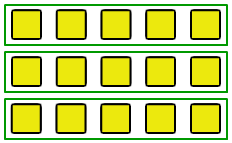# Division Groups

4,592 Plays
Rate 0 stars
Common Core
Quiz size:
Message preview:
Someone you know has shared quiz with you:

To play this quiz, click on the link below:

https://www.turtlediary.com/quiz/division-groups.html

Hope you have a good experience with this site and recommend to your friends too.

Login to rate activities and track progress.
Login to rate activities and track progress.

After division, all groups will have equal numbers of objects.

For example: Suppose we are given 15 squares and we need to make groups of 5 squares each.Let's see how many groups can we make.

For this, we divide them in groups of 5 squares each.There are 3 groups of 5.

So, the given 15 squares can be arranged in 3 groups of 5 squares each.

ds

A B C D E F G H I J K L M N O P Q R S T U V W X Y Z

### Help

##### Remember :

The smallest number is the one that comes first while counting.

##### Solution :

To arrange the given numbers in order from smallest to greatest, find the smallest number among all the given numbers.

21,27,23

21 is the smallest number.